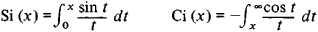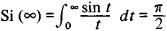# Sine Integral and Cosine Integral

## Sine Integral and Cosine Integral

special functions respectively defined by the integralsThese functions were introduced by the Italian mathematician L. Mascheroni in 1790. However it was already known (1781) to L. Euler thatThis integral is the simplest example of an improper integral that is convergent but not absolutely convergent. The functions Si (x) and Ci (x) are encountered in various problems of analysis and engineering, and comprehensive tables of them are available.

Site: Follow: Share:
Open / Close